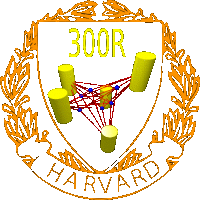Omni 3D project
Glossary 300R: Mathematics of 3D image synthesis
Glossary
Office: SciCtr 434
 Structure from motion problem : The structure from motion problem is an inverse problem in geometry: "From an image sequence taken by a camera undergoing unknown motion, extract the shape of the scene as well as the camera motion." This is the formulation of T. Kanade and D. Morris in Phil. Trans R. Soc. Lond, 1998, 356, 1153-1173. The term "structure from motion" was used first by Ullman in 1979 when he formulating his classical "structure from motion" theorem. The term is sometimes used in other contexts also like when the camera positions are known in multiple view geometry. The problem depends very much on the camera model and the dimension. It is already interesting in 2 dimensions where it can lead to interesting problems in planimetry. Assume we have n points and m cameras. Which configurations allow a unique reconstruction? If the reconstruction is not unique, what ambiguities can occur? We propose the following more mathematical definition: Given a manifold N of points and a manifold M of smooth maps Q on S such that Q(S) is diffeomorphic to a fixed manifold S. For n points Pi in N and m points in M, we are given the image data Qj(Pi) in S. The problem is to reconstruct both the points and the cameras, that is to invert the structure from motion map F: Nn x Mm -> Sn m modulo a global symmetry group G acting on NxM leaving the image data invariant. Structure from motion map : For m cameras and n points the structure from motion map is a map from Nn x Mm to Snm, where S is the retinal manifold. Cameras : A camera Q is a map on a manifold N such that the image of Q is a lower dimensional surface in N and so that Q2=Q. A camera manifold is a set of cameras which is a manifold and such that all images S=Q(N) are diffeomorphhic to a fixed manifold S. The retinal surface S is typically a hypersurface but can have lower dimensions. If N is a linear space and Q is an affine map, the camera is called affine. if Q is a perspective map, the camera Q is is called perspective. Projective cameras: is defined by a projective transformation of rank 3. The image is a plane. Perspective cameras: is defined as a central projection from a point, the center of projection to a plane. Affine cameras: is a projective camera, where the first 3 entries of the last row is zero. Geometrically it is a general affine transformation composed with a projection onto a plane. Orthographic cameras: the 3x3 matrix of the the first three columns contains two orthonormal vectors as row vectors. Geometrically it is an Euclidean transformation composed with an orthogonal projection onto a plane. Weakly perspective cameras: take an orthographic camera and compose it with a scaling. Geometrically it is a similiarity transformation composed with an orthogonal projection onto a plane. Spherical camera: defined by a point C and a sphere around C. A point is maped onto the sphere by intersectiing the line through P and C with the sphere. Camera parameter manifold : A finite dimensional manifold M which which parametrizes a class of cameras. It is assumed that each Q in M has the property that Q(N) is diffeomorphic to a fixed retinal manifold S. Examples: A general projective camera is parametrized by the space of 4x3 matrices of rank 3. An affine camera is parametrized by the space of all hyperplanes in space, and an Euclidean transformation in the plane which can be written as S2 x S1 x R2 = SO3 x R2. Global Camera symmetry group : A group G of transformations on the point camera space N x M which is an integral of the structure from motion map F. Given n points and m cameras taking pictures of these n points. A linear transformation in space belongs to the camera symmetry group G if applying this transformation to the points and cameras does not change the pictures taken by the cameras. Example: For an orthographic affine camera, the symmetry group is the Euclidean group, the subgroup of the affine group generated by rotations and translations. Collineation: An other word for an invertible projective transformation or homography. The set of all collineations forms a group, the projective group. Homography: An other word for an invertible projective transformation. The set of all homographies forms a group, the projective group. Chasles problem: Historically one of the first apperanaces of the structure from motion problem in mathematics. Chasles asks in 1855: we are given in the plane two sets of seven points in correspondence. For each set find a point in space such that the two sets of seven lines they define are related by a collineation. See Faugeras 1996, p. 256. (M. Chasles, Question no 296, Nouv. Ann. Math, 14:50, 1855)